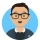Home
IT Knowledge
Inspiration
Languages
EN

# React - render first N elements from array

1 points
Created by:Krzysiek
351

In this article, we would like to show you how to render the first N elements from the array in React.

To get the first N number of elements from an array we can use the `slice()`,  then we convert the array items to React elements with `map()` method.

Quick solution:

``````  // some code here
let n = 3;   // number of n first elements to render
let newArray = array.slice(0, n).map(i => {
return <element key={i.id} />
};
return (
<div>
{newArray}
</div>
)
};``````

Note:

Remember that each element should have a unique key - it helps React optimally manage changes in the DOM. Such a key may be, for example, the id assigned to each element.

### Practical example:

``````// ONLINE-RUNNER:browser;

// Note: Uncomment import lines during working with JSX Compiler.
// import React from "react";
// import ReactDOM from "react-dom";

const App = () => {
const buttons = [
{ id: 1, text: "Button 1" },
{ id: 2, text: "Button 2" },
{ id: 3, text: "Button 3" },
{ id: 4, text: "Button 4" },
{ id: 5, text: "Button 5" }
];

// try to change n number
const n = 2;
const firstNElements = buttons.slice(0, n).map(({ id, text }) => {
return (<button key={id}>{text}</button>)
});

return (
<div>
<p>First {n} elements from array</p>
{firstNElements}
</div>
);
};

const root = document.querySelector('#root');
ReactDOM.render(<App />, root);``````